SSC  >  SSC CPO Mock Test (Paper 1) - 8

# SSC CPO Mock Test (Paper 1) - 8

Test Description

## 200 Questions MCQ Test SSC CPO & Constable - Mock Tests & Previous Year Papers | SSC CPO Mock Test (Paper 1) - 8

SSC CPO Mock Test (Paper 1) - 8 for SSC 2023 is part of SSC CPO & Constable - Mock Tests & Previous Year Papers preparation. The SSC CPO Mock Test (Paper 1) - 8 questions and answers have been prepared according to the SSC exam syllabus.The SSC CPO Mock Test (Paper 1) - 8 MCQs are made for SSC 2023 Exam. Find important definitions, questions, notes, meanings, examples, exercises, MCQs and online tests for SSC CPO Mock Test (Paper 1) - 8 below.
Solutions of SSC CPO Mock Test (Paper 1) - 8 questions in English are available as part of our SSC CPO & Constable - Mock Tests & Previous Year Papers for SSC & SSC CPO Mock Test (Paper 1) - 8 solutions in Hindi for SSC CPO & Constable - Mock Tests & Previous Year Papers course. Download more important topics, notes, lectures and mock test series for SSC Exam by signing up for free. Attempt SSC CPO Mock Test (Paper 1) - 8 | 200 questions in 120 minutes | Mock test for SSC preparation | Free important questions MCQ to study SSC CPO & Constable - Mock Tests & Previous Year Papers for SSC Exam | Download free PDF with solutions
 1 Crore+ students have signed up on EduRev. Have you?
SSC CPO Mock Test (Paper 1) - 8 - Question 1

### Complete the analogous pair.XYZ: CBA :: UVW :?

SSC CPO Mock Test (Paper 1) - 8 - Question 2

### Re-arrange the given words in alphabetical order and tick the one that comes in the middle.

SSC CPO Mock Test (Paper 1) - 8 - Question 3

### Find out which word or name will be in the middle after the words or names are arranged in the alphabetical order.

SSC CPO Mock Test (Paper 1) - 8 - Question 4
Choose the word which is least like the other words in the group .
SSC CPO Mock Test (Paper 1) - 8 - Question 5
Choose the group of letters which is different from others.
SSC CPO Mock Test (Paper 1) - 8 - Question 6
Complete the analogous pair.
Hill : Mountain : : Stream : ?
SSC CPO Mock Test (Paper 1) - 8 - Question 7
Choose the word which is least like the other words in the group .
Detailed Solution for SSC CPO Mock Test (Paper 1) - 8 - Question 7 All except Universe form a part of the universe
SSC CPO Mock Test (Paper 1) - 8 - Question 8
Complete the analogous pair.
Annihilation : Fire : : Cataclysm : ?
SSC CPO Mock Test (Paper 1) - 8 - Question 9
Complete the analogous pair.
War : Death : : Smoke :?
SSC CPO Mock Test (Paper 1) - 8 - Question 10
Complete the analogous pair.
Kannada : Karnataka :: ? Kerala
SSC CPO Mock Test (Paper 1) - 8 - Question 11
Ravi walks 100 m from his house towards North. From there he goes 100 m towards West. Here is the house of Sunder. From there they both goes to the market which is in the South-West direction from Sunder's house. If the market is in the West of Ravi's house, then how far is the market from Ravi's house?
SSC CPO Mock Test (Paper 1) - 8 - Question 12
If the following alphabets are arranged in the reverse order, which letter will be 8th letter to the left of 7th letter counting from the right end?
A B C D E F G H I J K L M N O P Q R S T U V W X Y Z
SSC CPO Mock Test (Paper 1) - 8 - Question 13
How many triangles are there in the following figure?
Detailed Solution for SSC CPO Mock Test (Paper 1) - 8 - Question 13 The figure may be labelled as shown in the following figure:

It is clear from the figure that there are fourteen triangles in the upper half of the figure
ABGE - EIJ, EFM, AFE, HFI, FOP, OBP, PBG, PGQ, PMQ, PFM, FBM, BMG, FMG and FEG
There are four triangles in the lower half of the figure EGCD - EMD, JMK, DKL and DMN
There are two triangles in the main figure ABCD - DBC, ADB
Hence, there are a total of twenty triangles in the figure.
SSC CPO Mock Test (Paper 1) - 8 - Question 14
Jai Prakash walked 40 metres facing towards North. From there he walked 50 metres after turning to his left. After this he walked 40 metres after turning to his left. How far and in what direction is he now from his starting point?
Detailed Solution for SSC CPO Mock Test (Paper 1) - 8 - Question 14
∴ AB = OC
∵ AB = 50 m
∴ OC = 50 m
∴ C is in West of O
SSC CPO Mock Test (Paper 1) - 8 - Question 15
Group the following figures into three classes on the basis of identical properties.
Detailed Solution for SSC CPO Mock Test (Paper 1) - 8 - Question 15 1,7,9 contain two similar elements one inside the other but not touching each other.
2,3,6 contain two similar elements one inside the other and both touching each ohter.
4,5,8 are divided into equal parts by straight lines emerging from the centre.
SSC CPO Mock Test (Paper 1) - 8 - Question 16

Find the missing character.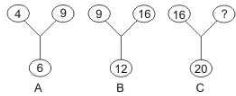Detailed Solution for SSC CPO Mock Test (Paper 1) - 8 - Question 16

The square of the number at the bottom is equal to the product of the two upper numbers. Thus,
In fig. (A), 4 x 9 = 6 2 = 36.
In fig. (B), 9 x 16 = 12 2 = 144.
Let the missing number in fog. (C) be x
Then 16 x x = 20 2 = 400 o r x = 400 16 = 25.

SSC CPO Mock Test (Paper 1) - 8 - Question 17
Find the missing character.
Detailed Solution for SSC CPO Mock Test (Paper 1) - 8 - Question 17 The second column, 22 + 2 - 23 = 1
In the third column, 40 + 5 - 43 = 2
∴ In the first column, missing number = 21 + 1 - 20 = 2
SSC CPO Mock Test (Paper 1) - 8 - Question 18
Find the missing character.
Detailed Solution for SSC CPO Mock Test (Paper 1) - 8 - Question 18 The two ends of each line segemnt contain a number and its square.
So, missing number = 12=1.
SSC CPO Mock Test (Paper 1) - 8 - Question 19
How many pairs of letters in the word 'DABBLE' have as many letters between them in the word as in the alphabet ?
Detailed Solution for SSC CPO Mock Test (Paper 1) - 8 - Question 19
$\frac{Left to Right}{A - B, A - E, B - E}$
$\frac{Right to Left}{B - D}$
There are four such pairs
SSC CPO Mock Test (Paper 1) - 8 - Question 20
Choose the odd one out.
Detailed Solution for SSC CPO Mock Test (Paper 1) - 8 - Question 20 There are two patterns
Pattern 1
All the consecutive pairs
YZ, PQ, EF, MN, JK
Pattern 2
Second alphabet is 2 alphabets away from the last
Hence, NMK is the odd one out
SSC CPO Mock Test (Paper 1) - 8 - Question 21
Choose the odd one out.
SSC CPO Mock Test (Paper 1) - 8 - Question 22

If 'M' means '÷', 'R' '+', 'T' means '-' and 'K' means 'x' then -
20 R 16 K 5 M 10 T 8 = ?

Detailed Solution for SSC CPO Mock Test (Paper 1) - 8 - Question 22

20 R 16 K 5 M 10 T 8
= 20 + 16 x 5 ÷ 10 - 8
= 20 + 16 x ( 1/ 2 ) - 8
= 20 + 8 - 8
= 20

SSC CPO Mock Test (Paper 1) - 8 - Question 23
In a certain code COIMBATORE is written as DPJNCBUPSF. How is INDORE written in that code?
Detailed Solution for SSC CPO Mock Test (Paper 1) - 8 - Question 23
SSC CPO Mock Test (Paper 1) - 8 - Question 24
If A stands for Addition , S stands for Subtraction, M stands for Multiplication, D stands for Division, G for Greater Than and L for Less than, Then which of the following alternatives will be logically correct.
SSC CPO Mock Test (Paper 1) - 8 - Question 25
Choose the odd one out.
SSC CPO Mock Test (Paper 1) - 8 - Question 26

If 'x' means '-', '+' means '÷', '-' means '+' and '÷' means 'x' then what is the value of 136 + 4 ÷ 5 - 68 x 75?

Detailed Solution for SSC CPO Mock Test (Paper 1) - 8 - Question 26

136 ÷ 4 x 5 + 68 - 75
= 34 x 5 - 7
⇒ 170 - 7 = 163

SSC CPO Mock Test (Paper 1) - 8 - Question 27
Choose the odd one out.
SSC CPO Mock Test (Paper 1) - 8 - Question 28
Choose the odd one out.
SSC CPO Mock Test (Paper 1) - 8 - Question 29
Choose the correct alternative that will continue the same pattern and fill in the blank.
13, 32, 24, 43, 35, (....), 46, 65, 57, 76
SSC CPO Mock Test (Paper 1) - 8 - Question 30
In each question, three sequences of letters/numerals are given which correspond to each other. Find out the letters/numerals that come in vacant places.
A _ B A C _ D _ B C D C
_ 3 _ 2 _ 1 _ 4 ? ? ? ?
d c _ _ b a c b _ _ _ _
SSC CPO Mock Test (Paper 1) - 8 - Question 31
Which one set of letters when sequentially placed at the gaps in the given letter series shall complete it?
cc_ccbc_accbcc_c_b
SSC CPO Mock Test (Paper 1) - 8 - Question 32
Choose the missing term out of the given alternatives.
SSC CPO Mock Test (Paper 1) - 8 - Question 33
Choose the missing term out of the given alternatives.
3F, 6G, 11I, 18L, ?
SSC CPO Mock Test (Paper 1) - 8 - Question 34
In each question, three sequences of letters/numerals are given which correspond to each other. Find out the letters/numerals that come in vacant places.
C _ B _ D _ A _ B B D D
2 _ _ 4 _ 3 4 _ ? ? ? ?
_ a _ c b a _ d _ _ _ _
SSC CPO Mock Test (Paper 1) - 8 - Question 35
In each question a statement followed by three assumptions numbered I, II and III is given. Consider the statement and decide which of the given assumption is implicit.
Statement : The scool authority decided to open a summer school this year in the school compound for the students in the age range of 7 - 14 years.
Assumptions : I. All the students will attend the summer school.
II. All the parents will prefer to remain in the city than going out of town for enabling their children to attend the summer school.
III. Those who cannot afford to go out of station will send their children to summer school.
SSC CPO Mock Test (Paper 1) - 8 - Question 36
If 'red means 'white', 'white' means 'black', 'black' means 'yellow', 'yellow' means 'green' and 'green' means 'blue' and 'blue means 'indigo'; then which of the following will represent the colour of sunflower?
SSC CPO Mock Test (Paper 1) - 8 - Question 37
Below is given statement followed by four conclusions numbered I, II, III and IV. You have to consider the statement and the following conclusions and decide which of the conclusions is follows in the statement :
Statements : a. All flowers are buses.
b. Some buses are cats.
c. All cats are tigers.
conclusions :
I. Some tigers are buses.
II. Some tigers are flowers.
III. Some cats are flowers.
IV. Some buses are tigers.
SSC CPO Mock Test (Paper 1) - 8 - Question 38
The Managing Director entered the conference room ten minutes before 12.30 hours for interviewing, He came 20, minutes before the chairman who was 30 minutes late. At what time the interviews were scheduled?
SSC CPO Mock Test (Paper 1) - 8 - Question 39
Which of the following words does not belong to the above set?
‘Gym, hymn, lynx, pygmy, rhythm’
SSC CPO Mock Test (Paper 1) - 8 - Question 40
How many meaningful English words can be made with the letters ALEP using each letter only once in each word?
Detailed Solution for SSC CPO Mock Test (Paper 1) - 8 - Question 40 Reqd. words are : LEAP, PLEA, PALE and PEAL
SSC CPO Mock Test (Paper 1) - 8 - Question 41
Consider the following statements about the megacities of India :
1. Population of each megacity is more than 5 millions .
2. All the megacities are important sea ports.
3. Megacities are either national or state capitals
Which of these statements are correct ?
SSC CPO Mock Test (Paper 1) - 8 - Question 42

In the following questions, select a figure from amongst the four alternatives, which when placed in the blank space of fig. (X) would complete the pattern.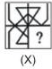SSC CPO Mock Test (Paper 1) - 8 - Question 43

In each question below is given a group of letters followed by four combinations of digits/symbols marked (A), (B), (C) and (D). You have to find which of the combinations correctly represents the groups of letters based on the following coding system and mark the letter of that combination as the answer. If none of the four combinations correctly represents the group of letters, mark (E) i.e. 'None of these' as the answer.
LetterACNDQVTGYRNumber Code7693850241LetterACNDQVTGYRNumber Code7693850241

NTRDQV

SSC CPO Mock Test (Paper 1) - 8 - Question 44

In each question below is given a group of letters followed by four combinations of digits/symbols marked (A), (B), (C) and (D). You have to find which of the combinations correctly represents the groups of letters based on the following coding system and mark the letter of that combination as the answer. If none of the four combinations correctly represents the group of letters, mark (E) i.e. 'None of these' as the answer.
LetterACNDQVTGYRNumber Code7693850241LetterACNDQVTGYRNumber Code7693850241

YRVAGC

SSC CPO Mock Test (Paper 1) - 8 - Question 45

In each of the following question, three classes of persons, objects or streams are given. Each class can be represented by one of the seven Venn diagrams given below. Indicate which figure best represents the relationship among three classes?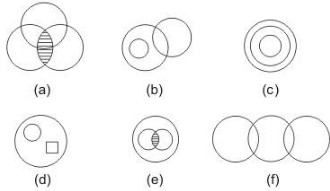Which of the following Venn diagrams best illustrates the three classes :
Smokers
Non-smokers
Artists.

Detailed Solution for SSC CPO Mock Test (Paper 1) - 8 - Question 45

Some Artists are Smokers, others are Non-smokers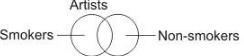SSC CPO Mock Test (Paper 1) - 8 - Question 46

In each of the following question, three classes of persons, objects or streams are given. Each class can be represented by one of the seven Venn diagrams given below. Indicate which figure best represents the relationship among three classes?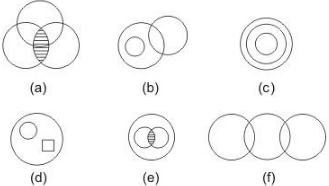Which of the following Venn diagrams best illustrates the three classes :
Mango
Banana
Seed

SSC CPO Mock Test (Paper 1) - 8 - Question 47

Complete the analogous pair.

31. 11 : 145 :: 14 : ?

Detailed Solution for SSC CPO Mock Test (Paper 1) - 8 - Question 47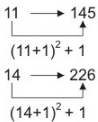SSC CPO Mock Test (Paper 1) - 8 - Question 48

Select the correct mirror image of the figure (X) from amongst the given alternatives.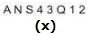SSC CPO Mock Test (Paper 1) - 8 - Question 49

Find out from amongst the four alternatives as to how the pattern would appear when the transparent sheet is folded at the dotted line.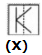SSC CPO Mock Test (Paper 1) - 8 - Question 50

Each of the following questions consists of five problem figures marked 1, 2, 3, 4, 5 followed by Answer figure marked A, B, C, D and E. Select a figure from the answer figures which will continue the same series as given in the problem figures.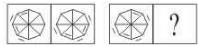SSC CPO Mock Test (Paper 1) - 8 - Question 51

In the questions, figure (X) is embedded in one of following figures. Find out the correct alternative.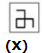SSC CPO Mock Test (Paper 1) - 8 - Question 52

Who of the following rejected the view that sovereignty is indivisible?

SSC CPO Mock Test (Paper 1) - 8 - Question 53

'Machiavelli's Prince was the first great work which marked a break away from the medieval way of thinking '.Who among this observation ?

SSC CPO Mock Test (Paper 1) - 8 - Question 54

Who is legally competent under the Indian Constitution to declare war or conclude peace?

SSC CPO Mock Test (Paper 1) - 8 - Question 55

India's first Prisoner's Bank was started at

SSC CPO Mock Test (Paper 1) - 8 - Question 56

On computers using Microsoft Windows XP, holding the Windows key down and pressing the letter E will:

SSC CPO Mock Test (Paper 1) - 8 - Question 57

There are ____________ bits in a byte.

SSC CPO Mock Test (Paper 1) - 8 - Question 58

Limited liability means :

SSC CPO Mock Test (Paper 1) - 8 - Question 59

A sustained and appreciable increase in the price level over a considerable period of time is known as;

SSC CPO Mock Test (Paper 1) - 8 - Question 60

If tea and coffee are substitutes, a reduction in the price of coffee will

SSC CPO Mock Test (Paper 1) - 8 - Question 61

The apex organisation in the field of rural credit is:

SSC CPO Mock Test (Paper 1) - 8 - Question 62

Which of the following States in India lead in the production of rice?

SSC CPO Mock Test (Paper 1) - 8 - Question 63

Tax changes in an economy form part of

SSC CPO Mock Test (Paper 1) - 8 - Question 64

An individual actual standard of living can be assessed by-

SSC CPO Mock Test (Paper 1) - 8 - Question 65

Fifty paisa coins are

SSC CPO Mock Test (Paper 1) - 8 - Question 66

Monsoon winds are :

SSC CPO Mock Test (Paper 1) - 8 - Question 67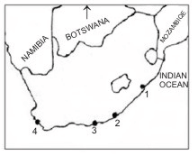In the map given above, four important towns of South Africa namely, A : Durban, B : Cape Town, C : East London and D : Port Elizabeth are marked with numbers. Match the city with the correct number and select the correct answer using the code given below.

SSC CPO Mock Test (Paper 1) - 8 - Question 68

Sun's total energy output intercepted by the earth is :

SSC CPO Mock Test (Paper 1) - 8 - Question 69

On which planet are scientists hopeful of the existence of some type of life?

SSC CPO Mock Test (Paper 1) - 8 - Question 70

What is hydrological cycle ?

SSC CPO Mock Test (Paper 1) - 8 - Question 71

The Battle of Plassey took place in

SSC CPO Mock Test (Paper 1) - 8 - Question 72

The premament settlement of Bengal was introduced by:

SSC CPO Mock Test (Paper 1) - 8 - Question 73

The Chola rulers patronised which language?

SSC CPO Mock Test (Paper 1) - 8 - Question 74

Bhana Bhata wrote

SSC CPO Mock Test (Paper 1) - 8 - Question 75

Gopuram is a characteristic of temples situated in -

SSC CPO Mock Test (Paper 1) - 8 - Question 76

With reference to human diet, which one of the following is an essential fatty acid?

SSC CPO Mock Test (Paper 1) - 8 - Question 77

Where in India is the Centre for DNA Finger Printing and Diagnostic located

SSC CPO Mock Test (Paper 1) - 8 - Question 78

In a bisexual flower, if androceium and gynaecium mature at different times, the phenomenon is known as

SSC CPO Mock Test (Paper 1) - 8 - Question 79

A girl is swimming on a swing in sitting position. When the same girl stands up, the period of swing will

SSC CPO Mock Test (Paper 1) - 8 - Question 80

A hunter aims his gum at a point between the eyebrows of a monkey sitting on a branch of a tree. Just as he fires, the monkey jumps down. The bullet will

Detailed Solution for SSC CPO Mock Test (Paper 1) - 8 - Question 80

As the trajectory of a projectile is parabolic, therefore, when the hunter aims at eyebrow of a monkey, it will shoot him after jumping downward

SSC CPO Mock Test (Paper 1) - 8 - Question 81

Consider the following statements:
1. Tapeworm is a hermaphrodite.
2. Round-worm has separate sexes.
3. Filaria is caused by a nematode.
4. Guinea-worm is an annelid.
Which of these are correct?

SSC CPO Mock Test (Paper 1) - 8 - Question 82

Optical fiber works on the principal of

SSC CPO Mock Test (Paper 1) - 8 - Question 83

Which one of the following is a vector quantity?

SSC CPO Mock Test (Paper 1) - 8 - Question 84

When one gene controls two or more different characters simultaneously, the phenomenon is called

SSC CPO Mock Test (Paper 1) - 8 - Question 85

A fuse is used in main electric supply as a safety device. Which one of the following statements about the fuse is correct?

SSC CPO Mock Test (Paper 1) - 8 - Question 86

Recently the Chief Minister of Odisha, Naveen Patnaik, released a book on his father titled ‘The Tall Man Biju Patnaik’. Who is the author of the book?

SSC CPO Mock Test (Paper 1) - 8 - Question 87

Combustion is the process in which

SSC CPO Mock Test (Paper 1) - 8 - Question 88

The meteorologist uses only his eyes to measure which of the following ?

SSC CPO Mock Test (Paper 1) - 8 - Question 89

Sun's heat reaches earth by which of the following modes of heat transmission ?

SSC CPO Mock Test (Paper 1) - 8 - Question 90

In summer, a cloudy night is hotter than a starlit night because

SSC CPO Mock Test (Paper 1) - 8 - Question 91

Cryogenic engines find applications in

SSC CPO Mock Test (Paper 1) - 8 - Question 92

"A poison would kill another poison" is the guiding philosophy of :

SSC CPO Mock Test (Paper 1) - 8 - Question 93

Why does radio perception improve slightly during the night ?

SSC CPO Mock Test (Paper 1) - 8 - Question 94

Thermos flask keeps a liquid hot because

SSC CPO Mock Test (Paper 1) - 8 - Question 95

In which the purest form of carbon is found ?

SSC CPO Mock Test (Paper 1) - 8 - Question 96

The main endocrine gland present in the human body is :

SSC CPO Mock Test (Paper 1) - 8 - Question 97

Which of the following international organisations has started the scheme 'Partnership for Peace' for a group of nations?

SSC CPO Mock Test (Paper 1) - 8 - Question 98

How is the new membership of United Naitons affected?

SSC CPO Mock Test (Paper 1) - 8 - Question 99

Which game is Ian Thorpe associated with:

SSC CPO Mock Test (Paper 1) - 8 - Question 100

Who has been appointed as the brand ambassador of the upcoming T20 Mumbai League?

SSC CPO Mock Test (Paper 1) - 8 - Question 101

Komal and Sunita solved a quadratic equation. In solving it, Komal made a mistake in the constant term and got the roots as 6 and 2, while Sunita made a mistake in the coefficient of x only and obtained the roots as -7 and -1. The correct roots of the equation are:

Detailed Solution for SSC CPO Mock Test (Paper 1) - 8 - Question 101

When there is no mistake in a and b, the sum of roots must be correct
When there is no mistake in a and c, the sum of roots must be correct
∴ Sum of roots = (6 + 2) = 8 & Product of roots = (- 7) (- 1) = 7
So, the correct equation is : x2 - 8x + 7 = 0
⇒ (x - 7) (x - 1) = 0
∴ The roots are 7, 1

SSC CPO Mock Test (Paper 1) - 8 - Question 102

The ages of A and B are in the ratio of 2:3. Ten yrs hence, the ratio will be 3:4. Their present ages are

Detailed Solution for SSC CPO Mock Test (Paper 1) - 8 - Question 102

Let the ages of A and B be 2x and 3x years respectively.
2x + 10 3x + 10 = 3 4
⇒ 4(2x + 10) = 3(3x + 10)
⇒ 8x + 40 = 9x + 30
⇒ 8x - 9x = 30 - 40
⇒ - x = - 10
⇒ x = 10
A's age = 2x = 2 x 10 = 20
B's age = 3x = 3 x 10 = 30

SSC CPO Mock Test (Paper 1) - 8 - Question 103

The solution set of the system of equations 4 x +5y=7 and 3 x +4y=5 is

Detailed Solution for SSC CPO Mock Test (Paper 1) - 8 - Question 103

4/ x+ 5y = 7 .....(i)

3 x + 4y = 5 .....(ii)
Multiplying equation (i) with 4 and 5 we get
16 /x + 20y = 28 .....(iii)
15 /x + 20y = 25 .....(iv)
Solving (iii) and (iv) we get
1 x = 3
x = 1 3
Put x = 1 3 . in equation (i) we get
12 + 5y = 7
or 5y = -5
or y = - 1
The Solution of equation is ( 1 3 , - 1)

SSC CPO Mock Test (Paper 1) - 8 - Question 104

A park is in form of a rectangle 120 m x 100m. At the centre of the park is a circular lawn. The area of the park excluding the lawn is 10614 m2. The cost of grass carpeting the lawn @ Rs. 27/m2 is

Detailed Solution for SSC CPO Mock Test (Paper 1) - 8 - Question 104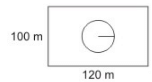Area of the park = 100 x 120
= 12000 m2
Area of the park excluding the lawn = 10614 m2
∴ The Area of the lawn = 12000 - 10614 = 1386 m2
The Cost of fencing the lawn = Rs. (27 x 1386) = Rs. 37422.00

SSC CPO Mock Test (Paper 1) - 8 - Question 105

The area of the sector of the circle is 1/10th the area of the circle. The angle of the sector is

Detailed Solution for SSC CPO Mock Test (Paper 1) - 8 - Question 105

Area of circle = A = πr2
Area of sectors of circle A = n 360 πr2
Where n = angle of the sector
According to question
n 360 πr2 = πr2 x 1 /10
⇒ n 360 = 1 10
⇒ 10n = 360
⇒ n = 360 10 = 36

SSC CPO Mock Test (Paper 1) - 8 - Question 106

The solution of 2x+3y=2 and 3x+2y=2 can be represented by a point in the coordinate planes in

SSC CPO Mock Test (Paper 1) - 8 - Question 107

What is the ratio of the areas of two circles whose radii are in the ratio 1:2 ?

Detailed Solution for SSC CPO Mock Test (Paper 1) - 8 - Question 107

Let radius of one circle is x
So radius of other is 2x
Ratio of area = πx 2 /π(2x) 2
= πx 2 /π4x 2 = 1 4 = 1 : 4

SSC CPO Mock Test (Paper 1) - 8 - Question 108

If 1 /x = 3 and y = 4, then what is x in terms of y?

Detailed Solution for SSC CPO Mock Test (Paper 1) - 8 - Question 108

1 /x = 3, y = 4, 1 = 4 /y

or x = 1/ 3 = 4 /3y

SSC CPO Mock Test (Paper 1) - 8 - Question 109

Sum of digits of the smallest number by which 1440 be multiplied so that it becomes a perfect cube,is

SSC CPO Mock Test (Paper 1) - 8 - Question 110

The area of triangle whose vertices are (1,-1), (-1,1) and (-1,-1) is

SSC CPO Mock Test (Paper 1) - 8 - Question 111

The average of the first nine prime numbers is:

Detailed Solution for SSC CPO Mock Test (Paper 1) - 8 - Question 111

Average = ( 2 + 3 + 5 + 7 + 11 + 13 + 17 + 19 + 23 9 ) = 100 9 = 11 1 9

SSC CPO Mock Test (Paper 1) - 8 - Question 112

A shopkeeper marks his goods at 40% above the cost price and allows a discount of 40% on the marked price. His loss or gain is

SSC CPO Mock Test (Paper 1) - 8 - Question 113

Difference between the Compound Interest and the simple interest accrued on an amount of Rs 18000, in 2 yrs was Rs 405. What was the rate of interest p.c.p.a?

Detailed Solution for SSC CPO Mock Test (Paper 1) - 8 - Question 113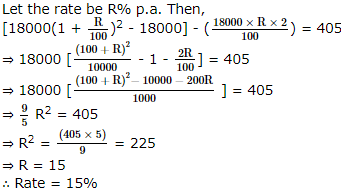SSC CPO Mock Test (Paper 1) - 8 - Question 114

The average of two numbers is 62. If 2 is added to the smaller number,the ratio between the numbers becomes 1:2. The smaller number is

SSC CPO Mock Test (Paper 1) - 8 - Question 115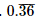expressed in the form of p/q equals

SSC CPO Mock Test (Paper 1) - 8 - Question 116

In the given figure of ∠AOB = 110º and ∠AOC is a right angle then measure of ∠BAC is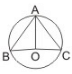SSC CPO Mock Test (Paper 1) - 8 - Question 117

Diagonals of quadrilateral are equal, perpendicular to each other and bisect each other. The quadrilateral is certainly a

SSC CPO Mock Test (Paper 1) - 8 - Question 118

AB and CD are two parallel chords of a circle. If AB = 10 cm, CD = 24 cm and distance between the chords is 17 cm then the radius of the circle is

SSC CPO Mock Test (Paper 1) - 8 - Question 119

An angles which is greater than 180º but less than 360º is called

SSC CPO Mock Test (Paper 1) - 8 - Question 120

The largest number which exactly divides 522,1276 and 1624 is

SSC CPO Mock Test (Paper 1) - 8 - Question 121

If a person standing on the bank of a river finds that the angle of the elevation of the top of a tower on the other bank directly opposite to him is 45º,then

SSC CPO Mock Test (Paper 1) - 8 - Question 122

The difference between two numbers is 7 and their sum is 35. What will be their product ?

SSC CPO Mock Test (Paper 1) - 8 - Question 123

In a certain shop,which stocks four types of caps, there are 1/3 as many red caps as blue and 1/2 as many green caps as red caps.There are equal numbers of green caps and yellow caps.If there are 42 blue caps,then what percent of the total caps in the shop are blue?

SSC CPO Mock Test (Paper 1) - 8 - Question 124

Three-fifth of a number is 30 more than 50% of that number. What is 80% of that number?

SSC CPO Mock Test (Paper 1) - 8 - Question 125

A cisten has a leak which would empty it in 8 hrs. If a tap is turned on, which admits 6 litres of water in a minute into the cistern which is now emptied in 12 hrs, then the capacity of the cistern is

Detailed Solution for SSC CPO Mock Test (Paper 1) - 8 - Question 125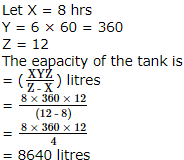SSC CPO Mock Test (Paper 1) - 8 - Question 126

A shopkeeper sold sarees at Rs.266 each after giving 5% discount on labelled price. Had he not given the discount, he would have earned a profit of 12% on the cost price. What was the cost price of each saree?

SSC CPO Mock Test (Paper 1) - 8 - Question 127

In Δ A B C , with usual notation, observe the two statements given below
I. r r 1 r 2 r 3 = Δ 2
II. r 1 r 2 + r 2 r 3 + r 3 r 1 = s 2
Which of the following is correct?

SSC CPO Mock Test (Paper 1) - 8 - Question 128

A students gets an aggregate of 60% marks in five subjects in the ratio 10 : 9 : 8 : 7 : 6. If the passing marks are 50% of the maximum marks and each subject has the same maximum marks, in how many subjects did he pass the examination?

SSC CPO Mock Test (Paper 1) - 8 - Question 129

Arun purchased a table at 3/5th of its labelled price and sold it for 14% more than its labelled price. What percentage of profit did Arun earn?

SSC CPO Mock Test (Paper 1) - 8 - Question 130

If m:n=3:2,then (4m+5n) :(4m-5n) is equal to

SSC CPO Mock Test (Paper 1) - 8 - Question 131

The harmonic mean of the roots of the equation (5 + √2)x2 - (4 + √5)s + 8 + 2√5 = 0 is

SSC CPO Mock Test (Paper 1) - 8 - Question 132

A sum fetched total simple interest of Rs 4016.25 at the rate of 9 p.c.p.a in 5 yrs. What is the sum?

SSC CPO Mock Test (Paper 1) - 8 - Question 133

Square root of 117649 is

SSC CPO Mock Test (Paper 1) - 8 - Question 134

The smallest value of θ satisfying √3(cotθ+tanθ)=4 is

SSC CPO Mock Test (Paper 1) - 8 - Question 135

10 men working 6 hrs a day can complete a work in 18 days. How many hrs a day must 15 men work to complete the same work in 12 days?

SSC CPO Mock Test (Paper 1) - 8 - Question 136

In a race of 200 m run, A beats S by 20 m and N by 40 m. If S and N are running a race of 100 m with exactly same speed as before then by how many metres will S beat N?

SSC CPO Mock Test (Paper 1) - 8 - Question 137

The value of 2 cos (x+π/3) is

SSC CPO Mock Test (Paper 1) - 8 - Question 138

If sinθ+cosθ=m and secθ+cosecθ=n, then n(m+1)(m-1)=

SSC CPO Mock Test (Paper 1) - 8 - Question 139

The length of longest pole that can be kept in a room 12 m long, 9 m broad and 8 m high, is .....

SSC CPO Mock Test (Paper 1) - 8 - Question 140

If both the radius and height of a right circular cone are increased by 20%, its volume will be increased by :

SSC CPO Mock Test (Paper 1) - 8 - Question 141

Find the surface area of a 10 cm x 4 cm x 3 cm brick.

SSC CPO Mock Test (Paper 1) - 8 - Question 142

Study the following graph carefully and answer the questions given below it.
Total value of Export of Rice, Sugar and Tea over the years
(In Rs Hundred crores)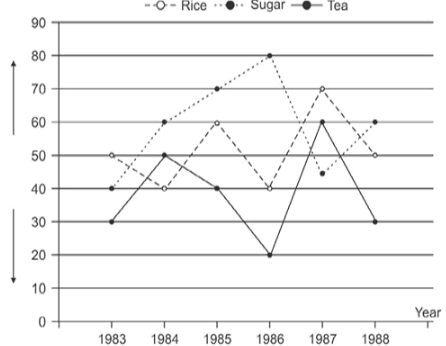In which year was the export value of tea maximum among the given years ?

SSC CPO Mock Test (Paper 1) - 8 - Question 143

Study the following graph carefully and answer the questions given below it.
Total value of Export of Rice, Sugar and Tea over the years
(In Rs Hundred crores)What was the difference in the export value of rice and tea in the year 1986 ?

SSC CPO Mock Test (Paper 1) - 8 - Question 144

Study the following graph carefully and answer the questions given below it.
Total value of Export of Rice, Sugar and Tea over the years
(In Rs Hundred crores)In which of the following years was the total export value of rice, tea and sugar together the minimum among the given years ?

Detailed Solution for SSC CPO Mock Test (Paper 1) - 8 - Question 144

In the year 1983 ⇒ Total export value (1983) = (3000 + 4000 + 5000) crore = 12000 crore

SSC CPO Mock Test (Paper 1) - 8 - Question 145

The gross investment of Life Insurance Corporation of India (in crore of rupees) in different sectors are shown in the pie chart given below. The letters denoting the various sectors are:
A = Private sector
B = Socially-oriented sector(Plan)
C = Socially-oriented sectors(Non-Plan)
D = Securities guranteed by the central government
E = State government securities
F = Central government securites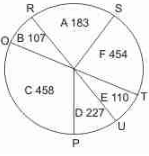The percentage of gross investments in State Government Securities is nearly:

SSC CPO Mock Test (Paper 1) - 8 - Question 146

The gross investment of Life Insurance Corporation of India (in crore of rupees) in different sectors are shown in the pie chart given below. The letters denoting the various sectors are:
A = Private sector
B = Socially-oriented sector(Plan)
C = Socially-oriented sectors(Non-Plan)
D = Securities guranteed by the central government
E = State government securities
F = Central government securitesThe central angle made by the sector indicating the investments made in the socially oriented sectors is nearly :

SSC CPO Mock Test (Paper 1) - 8 - Question 147

The gross investment of Life Insurance Corporation of India (in crore of rupees) in different sectors are shown in the pie chart given below. The letters denoting the various sectors are:
A = Private sector
B = Socially-oriented sector(Plan)
C = Socially-oriented sectors(Non-Plan)
D = Securities guranteed by the central government
E = State government securities
F = Central government securitesThe investment in Private sector is nearly how much percent higher than the investment in the State Government Securities?

SSC CPO Mock Test (Paper 1) - 8 - Question 148

The gross investment of Life Insurance Corporation of India (in crore of rupees) in different sectors are shown in the pie chart given below. The letters denoting the various sectors are:
A = Private sector
B = Socially-oriented sector(Plan)
C = Socially-oriented sectors(Non-Plan)
D = Securities guranteed by the central government
E = State government securities
F = Central government securitesThe ratio , of the area of the circle above ROU to the area of the circle below it is nearly :

SSC CPO Mock Test (Paper 1) - 8 - Question 149

The gross investment of Life Insurance Corporation of India (in crore of rupees) in different sectors are shown in the pie chart given below. The letters denoting the various sectors are:
A = Private sector
B = Socially-oriented sector(Plan)
C = Socially-oriented sectors(Non-Plan)
D = Securities guranteed by the central government
E = State government securities
F = Central government securitesThe investments in Socially oriented sectors (plan and non plan) is .... than the investement in the Government Securities (Central and the state ) by ....

Detailed Solution for SSC CPO Mock Test (Paper 1) - 8 - Question 149

Investment in socially oriented sector (Plan and Non Plan) = (107 + 458) = Rs 65 crores investment in central and State Government Securities = (454 + 110) = Rs 514 crores ∴ Difference = (565 - 564) = Rs 1 crore

SSC CPO Mock Test (Paper 1) - 8 - Question 150

Study the following graph carefully and answer the questions given below it.
Total value of Export of Rice, Sugar and Tea over the years
(In Rs Hundred crores)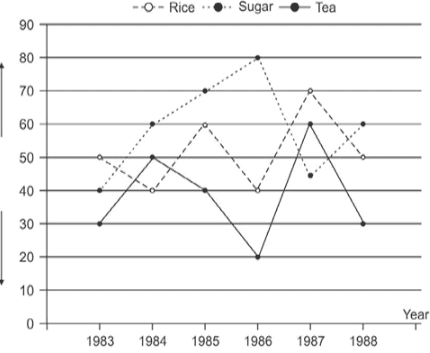In which of the following years was the total export value of rice, tea and sugar together the minimum among the given years ?

SSC CPO Mock Test (Paper 1) - 8 - Question 151

Find the Antonym of VINDICTIVE

SSC CPO Mock Test (Paper 1) - 8 - Question 152

Find the antonym of COMMEND

SSC CPO Mock Test (Paper 1) - 8 - Question 153

Find the antonym of LIBERAL

SSC CPO Mock Test (Paper 1) - 8 - Question 154

Choose the correctly spelt word.

SSC CPO Mock Test (Paper 1) - 8 - Question 155

Find the Antonym of TRIVIAL

SSC CPO Mock Test (Paper 1) - 8 - Question 156

Find the antonym of DESTINY

SSC CPO Mock Test (Paper 1) - 8 - Question 157

Choose the correctly spelt word.

SSC CPO Mock Test (Paper 1) - 8 - Question 158

Choose the correctly spelt word.

SSC CPO Mock Test (Paper 1) - 8 - Question 159

Choose the correctly spelt word.

SSC CPO Mock Test (Paper 1) - 8 - Question 160

Fill in the blank with appropriate word.
Ravi's behaviour is worthy of....by all the youngsters.

SSC CPO Mock Test (Paper 1) - 8 - Question 161

Fill in the blank with appropriate word.
.......eye witnesses, the news reporter gave a graphic description of how the fire broke.

SSC CPO Mock Test (Paper 1) - 8 - Question 162

Choose the correctly spelt word.

SSC CPO Mock Test (Paper 1) - 8 - Question 163

Fill in the blank with appropriate word.
Will you, like the........gentleman and solider you are, leave at once before he finds you here?

SSC CPO Mock Test (Paper 1) - 8 - Question 164

Fill in the blank with appropriate word.
Would you mind.........to the Principal how the trouble started?

SSC CPO Mock Test (Paper 1) - 8 - Question 165

Fill in the blank with appropriate word.
Nobody can say what.......him to commit this crime.

SSC CPO Mock Test (Paper 1) - 8 - Question 166

Choose the one which can be substituted for the given words/sentences :
One who has suddenly gained new wealth, power or prestige

SSC CPO Mock Test (Paper 1) - 8 - Question 167

Choose the one which can be substituted for the given words/sentences :
Indifference to pleasure or pain